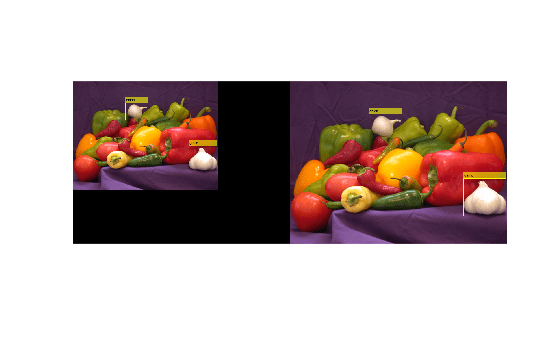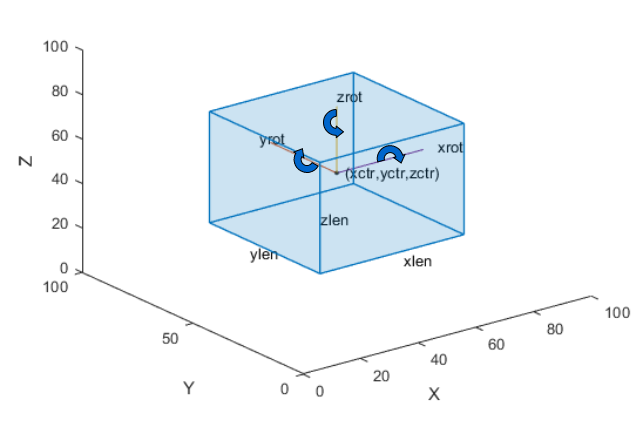# bboxresize

Resize bounding boxes

## Syntax

``bboxB = bboxresize(bboxA,scale)``

## Description

example

````bboxB = bboxresize(bboxA,scale)` resizes bounding boxes in `bboxA` by the amount specified by `scale`.```

## Examples

collapse all

`I = imread('peppers.png');`

Define bounding boxes and labels.

```bboxA = [ 410 230 100 90 186 78 80 60 ]```
```bboxA = 2×4 410 230 100 90 186 78 80 60 ```
```labelsA = [ "garlic" "onion" ];```

Resize the image and the bounding boxes.

```scale = 1.5; J = imresize(I,scale); bboxB = bboxresize(bboxA,scale); ```

Display the results.

```figure I = insertObjectAnnotation(I,'Rectangle',bboxA,labelsA); J = insertObjectAnnotation(J,'Rectangle',bboxB,labelsA); imshowpair(I,J,'montage')```## Input Arguments

collapse all

Bounding boxes, specified as an M-by-4, M-by-5, or M-by-9 nonsparse numeric matrix of M bounding boxes. Each row, M, of the matrix defines a bounding box as either an axis-aligned rectangle, a rotate rectangle, or a cuboid. The table below describes the format of the bounding boxes.

Bounding BoxDescription
Axis-aligned rectangle

Defined in pixel coordinates as an M-by-4 numeric matrix with rows of the form [x y w h], where:

• M is the number of axis-aligned rectangles.

• x and `y` specify the upper-left corner of the rectangle.

• w specifies the width of the rectangle, which is its length along the x-axis.

• h specifies the height of the rectangle, which is its length along the y-axis.

Rotated rectangle

Defined in spatial coordinates as an M-by-5 numeric matrix with rows of the form [xctr yctr xlen ylen yaw], where:

• M is the number of rotated rectangles.

• xctr and yctr specify the center of the rectangle.

• xlen specifies the width of the rectangle, which is its length along the x-axis before rotation.

• ylen specifies the height of the rectangle, which is its length along the y-axis before rotation.

• yaw specifies the rotation angle in degrees. The rotation is clockwise-positive around the center of the bounding box.Cuboid

Defined in spatial coordinates as an M-by-9 numeric matrix with rows of the form [xctr yctr zctr xlen ylen zlen xrot yrot zrot], where:

• M is the number of cuboids.

• xctr, yctr, and zctr specify the center of the cuboid.

• xlen, ylen, and zlen specify the length of the cuboid along the x-axis, y-axis, and z-axis, respectively, before rotation.

• xrot, yrot, and zrot specify the rotation angles of the cuboid around the x-axis, y-axis, and z-axis, respectively. The xrot, yrot, and zrot rotation angles are in degrees about the cuboid center. Each rotation is clockwise-positive with respect to the positive direction of the associated spatial axis. The function computes rotation matrices assuming `ZYX` order Euler angles [xrot yrot zrot].

The figure shows how these values determine the position of a cuboid.Scale, specified as a scalar or a row vector. When you specify a scalar, the function applies the same scale factor to the height and width of the bounding boxes in `bboxA`. When you specify a row vector, the function applies the factor in the first element of the vector to resize the height and the second element to resize the width of the bounding boxes.

## Output Arguments

collapse all

Warped bounding boxes, returned as an M2-by-N matrix of M2 bounding boxes. The number of bounding boxes returned is less than the number of bounding boxes in the input. Each row, M2, of the matrix defines one bounding box of the same type as the input `bboxA`.